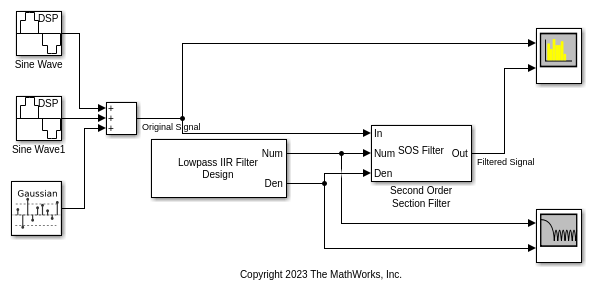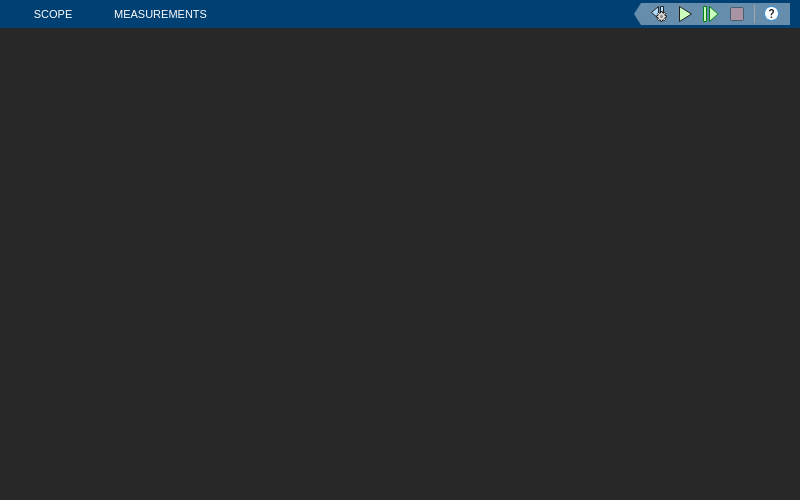# Tunable Lowpass IIR Filter

Design lowpass IIR filter with tunable filter specifications

Since R2023b

•Libraries:
DSP System Toolbox / Filtering / Filter Sources

## Description

Use the Tunable Lowpass IIR Filter block to design a lowpass IIR filter using the Butterworth, Chebyshev Type I and the Chebyshev Type II design methods. You can tune the filter design specifications such as the filter order, 3-dB cutoff frequency, passband ripple, and the stopband attenuation during simulation. When the values of these parameters change, the block redesigns the filter and outputs the numerator and denominator coefficients in the second-order section (SOS) form.

## Examples

expand all

Filter a noisy sinusoidal signal using the Second-Order Section Filter block. Obtain the numerator and denominator coefficients of the SOS filter using the Tunable Lowpass IIR Filter block.

Tune the frequency specifications of the SOS filter during simulation.

Open and Run Model

Open the secondordersectionfilter_lowpass model by clicking the Open Model button.

The input signal in the model is a sum of two sine waves with the frequencies of 100 Hz and 350 Hz. The sample rate is 1000 Hz and the number of samples in each frame is 1024. The Random Source block adds zero-mean white Gaussian noise with a variance of 1e-4 to the sum of the sine waves.

The Tunable Lowpass IIR Filter block designs a sixth-order lowpass IIR filter with the 3-dB cutoff frequency of 0.25rad/sample. The block generates coefficients as a cascade of second-order sections. Visualize the frequency response of the filter using the Filter Visualizer.

Run the model.

The Second-Order Section Filter block filters the noisy sinusoidal signal and attenuates the second tone at 350 Hz. Visualize the original sinusoidal signal and the filtered signal using the Spectrum Analyzer.Tune Frequency Specifications of SOS Filter

During simulation, you can tune the frequency specifications of the SOS filter by tuning the frequency parameters in the Tunable Lowpass IIR Filter block. The frequency response of the SOS filter updates accordingly.

Change the 3-dB cutoff frequency to 0.75rad/sample in the Tunable Lowpass IIR Filter block. The second tone of the sinusoidal signal is now unaffected as it now falls in the passband region of the filter.## Ports

### Input

expand all

Specify the filter order as a positive integer. You can change the filter order you input through this port during simulation.

#### Dependencies

To enable this port, select the Specify filter order from input port parameter.

Data Types: single | double | int8 | int16 | int32 | int64 | uint8 | uint16 | uint32 | uint64

Specify the 3-dB cutoff frequency of the filter in the normalized frequency units as a positive scalar less than or equal to 1. You can change the 3-dB cutoff frequency you input through this port during simulation.

#### Dependencies

To enable this port, select the Specify 3-dB cutoff frequency from input port parameter.

Data Types: single | double

Specify the passband ripple of the Chebyshev Type I filter as a positive scalar in dB. You can change the passband ripple you input through this port during simulation.

#### Dependencies

To enable this port:

• Set the Design method parameter to Chebyshev Type I.

• Select the Specify the passband ripple from input port parameter.

Data Types: single | double

Specify the stopband attenuation of the Chebyshev Type II filter as a positive scalar in dB. You can change the stopband attenuation you input through this port during simulation.

#### Dependencies

To enable this port:

• Set the Design method parameter to Chebyshev Type II.

• Select the Specify the stopband attenuation from input port parameter.

Data Types: single | double

### Output

expand all

Numerator coefficients, returned as a P-by-3 matrix (SOS form), where P is the maximum number of filter sections.

$b=\left[\begin{array}{ccc}{b}_{01}& {b}_{11}& {b}_{21}\\ {b}_{02}& {b}_{12}& {b}_{22}\\ ⋮& ⋮& ⋮\\ {b}_{0P}& {b}_{1P}& {b}_{2P}\end{array}\right]$

This equation represents the SOS filter in the transfer function form.

$H\left(z\right)=\prod _{k=1}^{P}{H}_{k}\left(z\right)=\prod _{k=1}^{P}\frac{{b}_{0k}+{b}_{1k}{z}^{-1}+{b}_{2k}{z}^{-2}}{{a}_{0k}+{a}_{1k}{z}^{-1}+{a}_{2k}{z}^{-2}},$

where

• b is a matrix of numerator coefficients.

• a is a matrix of denominator coefficients that the block outputs at the Den output port.

• k is the row index.

P equals ceil(Nmax/2), where Nmax is the value of the Filter maximum order parameter.

When the actual filter order N is less than the maximum filter order Nmax, the last ceil(Nmax/2) − ceil(N/2) sections are trivial with coefficients [b0, b1, b2] = [1, 0, 0].

When the actual filter order N is odd, the last nontrivial filter section has the coefficients [b0, b1, 0] = [1, 1, 0].

The data type of this port depends on the value of the Output data type parameter.

Data Types: single | double

Denominator coefficients, returned as a P-by-3 matrix (SOS form), where P is the maximum number of filter sections.

$a=\left[\begin{array}{ccc}{a}_{01}& {a}_{11}& {a}_{21}\\ {a}_{02}& {a}_{12}& {a}_{22}\\ ⋮& ⋮& ⋮\\ {a}_{0P}& {a}_{1P}& {a}_{2P}\end{array}\right]$

This equation represents the SOS filter in the transfer function form.

$H\left(z\right)=\prod _{k=1}^{P}{H}_{k}\left(z\right)=\prod _{k=1}^{P}\frac{{b}_{0k}+{b}_{1k}{z}^{-1}+{b}_{2k}{z}^{-2}}{{a}_{0k}+{a}_{1k}{z}^{-1}+{a}_{2k}{z}^{-2}},$

where

• a is a matrix of denominator coefficients and the leading denominator coefficient a0 is always 1.

• b is a matrix of numerator coefficients that the block outputs at the Num port.

• k is the row index.

P equals ceil(Nmax/2), where Nmax is the value of the Filter maximum order parameter.

When the actual filter order N is less than the maximum filter order Nmax, the last ceil(Nmax/2) − ceil(N/2) sections are trivial with coefficients [a0, a1, a2] = [1, 0, 0].

When the actual filter order N is odd, the last nontrivial filter section has the coefficients [1, a1, 0] = [1, 1, 0].

The data type of this port depends on the value of the Output data type parameter.

Data Types: single | double

Scale values for each second-order section, returned as a vector with P + 1 elements, where P is the maximum number of filter sections and equals ceil(Nmax/2), where Nmax is the value of the Filter maximum order parameter.

Tunable: Yes

#### Dependencies

To enable this port, select the Design has scale values parameter.

Data Types: single | double

## Parameters

expand all

Specify the filter design method as one of these:

• Butterworth

• Chebyshev Type I

• Chebyshev Type II

Select this parameter to specify the filter order from the input port N. When you clear this parameter, you can specify the filter order in the block dialog box using the Filter order parameter.

Specify the filter order as a positive integer less than or equal to the value of the Filter maximum order parameter.

Tunable: Yes

#### Dependencies

To enable this parameter, clear the Specify filter order from input port parameter.

Data Types: single | double | int8 | int16 | int32 | int64 | uint8 | uint16 | uint32 | uint64

Specify the maximum order of the filter as a positive integer. The value you specify in the Filter order parameter must be less than or equal to the value you specify in the Filter maximum order parameter.

Data Types: single | double | int8 | int16 | int32 | int64 | uint8 | uint16 | uint32 | uint64

Specify if the filter has scale values for each section. When you select this parameter, the block outputs the scale values through the g output port.

Select this parameter to specify the 3-dB filter cutoff frequency from the input port Fc. When you clear this parameter, you can specify the cutoff frequency in the block dialog box using the 3-dB cutoff frequency parameter.

Specify the 3-dB cutoff frequency of the filter in the normalized frequency units as a positive scalar less than or equal to 1.

Tunable: Yes

#### Dependencies

To enable this parameter, clear the Specify 3-dB cutoff frequency from input port parameter.

Data Types: single | double

Select this parameter to specify the passband ripple from the input port Ap. When you clear this parameter, you can specify the passband ripple in the block dialog box using the Passband ripple (dB) parameter.

#### Dependencies

To enable this parameter, set the Design method parameter to Chebyshev Type I.

Specify the passband ripple of the Chebyshev Type I filter as a positive scalar in dB.

Tunable: Yes

#### Dependencies

To enable this parameter:

• Set the Design method parameter to Chebyshev Type I.

• Clear the Specify the passband ripple from input port parameter.

Data Types: single | double

Select this parameter to specify the stopband attenuation from the input port Ast. When you clear this parameter, you can specify the stopband attenuation in the block dialog box using the Stopband attenuation (dB) parameter.

#### Dependencies

To enable this parameter, set the Design method parameter to Chebyshev Type II.

Specify the stopband attenuation of the Chebyshev Type II filter as a positive scalar in dB.

Tunable: Yes

#### Dependencies

To enable this parameter:

• Set the Design method parameter to Chebyshev Type II.

• Clear the Specify the stopband attenuation from input port parameter.

Data Types: single | double

Specify the sample time as -1 (inherited), Inf (constant sample time), or a positive scalar. This parameter determines when the block produces the output and updates its internal state during simulation. For more details, see What Is Sample Time? (Simulink).

Data Types: single | double

Specify the data type of the filter coefficients that the block outputs through the Num and Den ports. You can set the data type to double or single.

Specify the type of simulation to run as one of the following:

• Code generation –– Simulate model using generated C code. The first time you run a simulation, Simulink® generates C code for the block. Simulink reuses the C code in subsequent simulations as long as the model does not change. This option requires additional startup time but subsequent simulations are faster compared to Interpreted execution.

• Interpreted execution –– Simulate model using the MATLAB®  interpreter. This option shortens startup time but subsequent simulations are slower compared to Code generation.

## Block Characteristics

 Data Types double | integer | single Direct Feedthrough no Multidimensional Signals no Variable-Size Signals no Zero-Crossing Detection no

## Version History

Introduced in R2023b